Select Category
Select Category
Select Category

#### Options

Rs. 152.00 - Rs. 156.00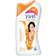Glycerin + honey
Rs. 160.00 Rs. 156.00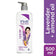Lavender+Almond oil
Rs. 160.00 Rs. 152.00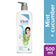Mint+Cucumber
Rs. 160.00 Rs. 152.00
Rs. 135.00 - Rs. 360.00100 ML
Rs. 135.00400 ML
Rs. 360.00
Rs. 10.00 - Rs. 340.00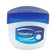85 g
Rs. 120.00 Rs. 117.00200 g
Rs. 340.00 Rs. 323.0012 g
Rs. 10.0021 g
Rs. 42.0042 g
Rs. 75.00250 g
Rs. 340.00
Rs. 10.00 - Rs. 399.0020 ML
Rs. 10.00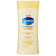40 ML
Rs. 49.00100 ML
Rs. 75.00200 ML
Rs. 199.00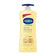400 ML
Rs. 325.00600 ML
Rs. 399.00
Rs. 99.00 - Rs. 375.00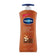200 Ml
Rs. 190.00400 Ml
Rs. 375.00100 Ml
Rs. 99.00
Rs. 95.00 - Rs. 335.00100 ML
Rs. 95.00400 ML
Rs. 345.00 Rs. 335.00
Rs. 99.00 - Rs. 360.00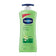200 ml
Rs. 225.00400ml
Rs. 360.00100 ml
Rs. 99.00
Rs. 20.00 - Rs. 328.00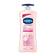40 ML
Rs. 49.00200ml
Rs. 210.00 Rs. 200.00400ml
Rs. 345.00 Rs. 328.0020ml
Rs. 20.00100 ML
Rs. 99.00
Rs. 155.00 - Rs. 410.00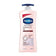100ml
Rs. 155.00200ml
Rs. 225.00 Rs. 214.00400ml
Rs. 410.00
Rs. 136.00 - Rs. 295.00100 ml
Rs. 140.00 Rs. 136.00200 ml
Rs. 310.00 Rs. 295.00
Rs. 79.00 - Rs. 299.00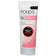50 g
Rs. 79.00100 g
Rs. 149.00200 g
Rs. 299.00
Rs. 67.00 - Rs. 242.00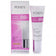9 G
Rs. 67.0018 G
Rs. 120.0030 G
Rs. 242.00
Rs. 156.00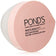Default Title
Rs. 160.00 Rs. 156.00
Rs. 85.00Default Title
Rs. 87.00 Rs. 85.00
Rs. 149.00 - Rs. 285.00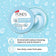75 g
Rs. 149.00147 g
Rs. 299.00 Rs. 285.00
Rs. 194.00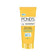Default Title
Rs. 199.00 Rs. 194.00
Rs. 110.00 - Rs. 280.00100 g
Rs. 110.00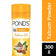300 g
Rs. 280.00
Rs. 60.00 - Rs. 310.0050 gm
Rs. 60.00100 gm
Rs. 110.00300 gm
Rs. 310.00
Rs. 10.00 - Rs. 310.00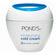30 Ml
Rs. 46.0055 Ml
Rs. 89.00100 Ml
Rs. 140.00200 Ml
Rs. 310.0014 g
Rs. 10.00
Rs. 262.00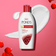Default Title
Rs. 270.00 Rs. 262.00
Items 1 to 20 of 30 total
• 1
• 2

## Contact Us if you need any help

The cookie settings on this website are set to 'allow all cookies' to give you the very best experience. Please click Accept Cookies to continue to use the site.

×